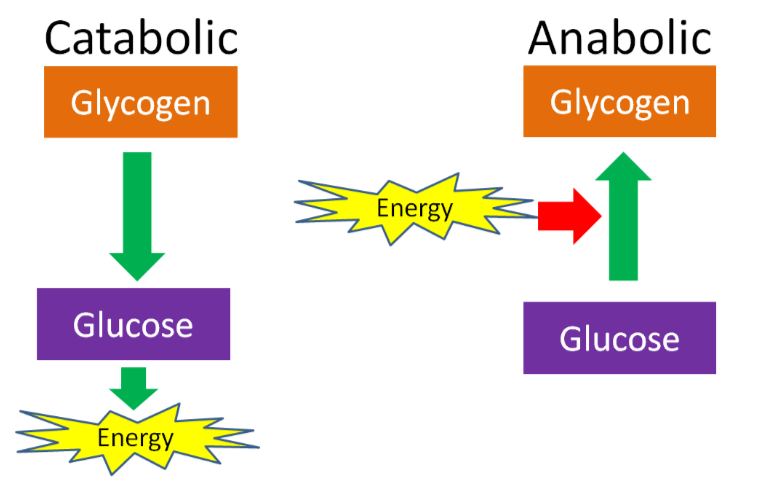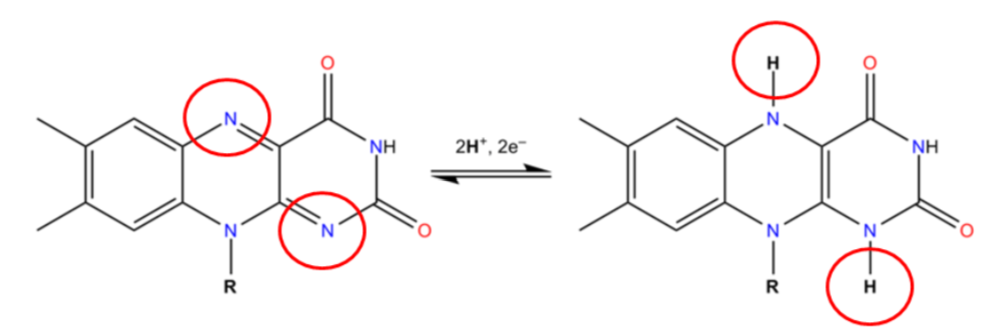# 6.1: Metabolism Basics

$$\newcommand{\vecs}{\overset { \rightharpoonup} {\mathbf{#1}} }$$ $$\newcommand{\vecd}{\overset{-\!-\!\rightharpoonup}{\vphantom{a}\smash {#1}}}$$$$\newcommand{\id}{\mathrm{id}}$$ $$\newcommand{\Span}{\mathrm{span}}$$ $$\newcommand{\kernel}{\mathrm{null}\,}$$ $$\newcommand{\range}{\mathrm{range}\,}$$ $$\newcommand{\RealPart}{\mathrm{Re}}$$ $$\newcommand{\ImaginaryPart}{\mathrm{Im}}$$ $$\newcommand{\Argument}{\mathrm{Arg}}$$ $$\newcommand{\norm}{\| #1 \|}$$ $$\newcommand{\inner}{\langle #1, #2 \rangle}$$ $$\newcommand{\Span}{\mathrm{span}}$$ $$\newcommand{\id}{\mathrm{id}}$$ $$\newcommand{\Span}{\mathrm{span}}$$ $$\newcommand{\kernel}{\mathrm{null}\,}$$ $$\newcommand{\range}{\mathrm{range}\,}$$ $$\newcommand{\RealPart}{\mathrm{Re}}$$ $$\newcommand{\ImaginaryPart}{\mathrm{Im}}$$ $$\newcommand{\Argument}{\mathrm{Arg}}$$ $$\newcommand{\norm}{\| #1 \|}$$ $$\newcommand{\inner}{\langle #1, #2 \rangle}$$ $$\newcommand{\Span}{\mathrm{span}}$$$$\newcommand{\AA}{\unicode[.8,0]{x212B}}$$

Metabolism consists of all the chemical processes that occur in living cells. These processes/reactions can generally be classified as either anabolic or catabolic. Anabolic means to build, catabolic means to breakdown. If you have trouble remembering the difference between the two, remember that anabolic steroids are what are used to build enormous muscle mass.Figure $$\PageIndex{1}$$: One of these two is taking anabolic steroids, which one would be your guess?

An anabolic reaction/pathway requires energy to build something. A catabolic reaction/pathway generates energy by breaking down something. This is shown in the example below of glucose and glycogen. The same is true for other macronutrients.Figure $$\PageIndex{2}$$: The breakdown of glycogen to glucose is catabolic. The glucose can then be used to produce energy. The synthesis of glycogen from glucose is anabolic and requires energy.

Anabolic and catabolic can also be used to describe conditions in the body. For instance, after a meal there is often a positive energy balance, or there is more energy and macronutrients than the body needs at that time. Thus, some energy needs to be stored and the macronutrients will be used for synthesis, such as amino acids being used for protein synthesis. However, after a fast, or a prolonged period without energy intake, the body is in negative energy balance and is considered catabolic. In this condition, macronutrients will be mobilized from their stores to be used to generate energy. For example, if prolonged enough, protein can be broken down, then the released amino acids can be broken down to be used as an energy source.

Query $$\PageIndex{1}$$

A number of the metabolic reactions oxidize or reduce compounds. A compound that is oxidized loses at least one electron, while a compound that is reduced gains at least 1 electron. To remember the difference, a mnemonic device such as OIL (oxidation is lost), RIG (reduction is gained) is helpful. Oxidation-reduction reactions are illustrated in the figure below.Figure $$\PageIndex{3}$$: The purple compound is being oxidized, the orange compound is being reduced1

Another way to remember oxidation versus reduction is LEO goes GER (like a lion)

Lose Electrons = Oxidize

Gain Electrons = Reduce

Iron is a good example we can use to illustrate oxidation-reduction reactions. Iron commonly exists in two oxidation states ($$\ce{Fe^3+}$$ or $$\ce{Fe^2+}$$). It is constantly oxidized/reduced back and forth between the two states. The oxidation/reduction of iron is shown below.

$$\ce{Fe^3+ + e- → Fe^2+ Reduced}$$

$$\ce{Fe^2+ → Fe^3+ + e- Oxidized}$$

However, some oxidation reduction reactions are not as easy to recognize. There are some simple rules to help you recognize less obvious oxidation/reduction reactions that are based upon the gain or loss of oxygen or hydrogen. These are as follows:

• Oxidation: gains oxygen or loses hydrogen
• Reduction: loses oxygen or gains hydrogen

Query $$\PageIndex{2}$$

## Cofactors

A number of enzymes require cofactors to function. Some also require what other textbooks and resources refer to as coenzymes. But to keep things simple, we are going to include these coenzymes in our definition of cofactors. Thus, cofactors can be either organic or inorganic molecules that are required by enzymes to function. Many organic cofactors are vitamins or molecules derived from vitamins. Most inorganic cofactors are minerals. The reason why some vitamins and minerals are essential nutrients is because of their required role as cofactors for some enzymes. Cofactors can be oxidized or reduced for the enzymes to catalyze the reactions.

Two common cofactors that are derived from the B vitamins, niacin and riboflavin, are nicotinamide adenine dinucleotide ($$\ce{NAD}$$) and flavin adenine dinucleotide ($$\ce{FAD}$$), respectively. The structure of $$\ce{NAD}$$ and $$\ce{FAD}$$ are shown below.Figure $$\PageIndex{6}$$Figure $$\PageIndex{7}$$

Both of these cofactors can be reduced (or oxidized as well); $$\ce{NAD}$$ is reduced to form $$\ce{NADH}$$, while $$\ce{FAD}$$ is reduced to form $$\ce{FADH2}$$ as shown in the 2 figures below.Figure $$\PageIndex{6}$$: The reduction of $$\ce{NAD}$$ to form $$\ce{NADH}$$4Figure $$\PageIndex{7}$$: The reduction of $$\ce{FAD}$$5 to $$\ce{FADH2}$$

An example of a mineral that serves as a cofactor is $$\ce{Fe^2+}$$ for proline and lysyl hydroxylases. We will discuss later in detail why vitamin C (ascorbic acid) is needed to reduce iron to $$\ce{Fe^2+}$$ so that it can serve as a cofactor for proline and lysyl hydroxylases.Figure $$\PageIndex{8}$$: Iron ($$\ce{Fe^2+}$$) is a cofactor for proline and lysyl hydroxylases

Query $$\PageIndex{3}$$

## References

1. en.Wikipedia.org/wiki/Image:G...e_Platform.jpg